Invalidation of the Inverse Square Law for

Spherical Light Emitting Objects

Karunakar Marasakatla

(Updated: 08/22/11)

(www.kmarasakatla.com)

Abstract

In addition to the brightness of a light emitting (active) object and the distance between the active and passive objects; the amount of light reaches a passive object also depends upon the amount of the surface area of the active object exposed towards the passive object. Inverse square law of distance for light is not valid in instances where the light emitting objects are in different shapes such as sphere (star) or cylinder (florescent  tube); in which case the surface area of these objects exposed to an external object varies with the size of the objects.

Introduction

Assume the source of light as the spots of a contrast color on the surface of an inflated balloon. When the balloon is deflated to half of its original size, not only the brightness of the spots will increase but also more number of spots will be visible if we view the balloon from the same distance to the center of the balloon. If our star, the sun, collapses to half of its present size, an increased amount of surface area of the sun will be exposed towards the earth, resulting in the increase in the total amount of light reaches the earth. This is a clear violation of the inverse square law (ISL) for light.

Before delving into the details of the ISL, lets first look at some of the basic laws about the light and its propagation. We can summarize two of the basic properties of light and its propagation in the following points.

• A glowing object is a collection of point size light emitting objects (LEOs). The surface of the sun can be assumed as having a collection of N million light bulbs spread uniformly all over its surface. The number of light bulbs in a fixed percentage of the surface area of the LEO, irrespective of its radius, will always remain the same. One quarter or 25% of the surface area will have N/4 number of light bulbs.
• The light emanating from the opposite side of the sun will never reach the earth. If the other hemisphere of the sun also faces towards the earth then the amount of light reaches the earth from the sun will double. It means the amount of light reaches a passive object depends upon the percentage of the surface area of the light emitting object exposed towards the passive object. Number of point size LEOs facing the passive object will increase with the increase in the surface area of the LEO exposed towards the passive object.

In the derivation of inverse square law of distance for light, it was always assumed that a constant percentage of the surface area of the LEO will be facing towards the passive object irrespective of the size of the objects and the distance between them. A close analysis of the surface areas exposed between two spherical objects reveals that the percentage of the surface area of a spherical LEO exposed towards another spherical object increases with the decrease in the radius of the light emitting object.

Exposed Surface Area of a Spherical LEO towards another Spherical Object

Suppose two spherical objects A and B, A being the active object and the B as a passive object, facing each other as shown in Fig 1. In this case, it is important to note that the light emits from the surface of the sphere instead of from the center of the object.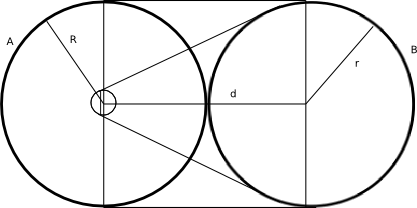Fig 1. Exposure of surface area between two spheres.

The equation for the surface area of the sphere A exposed towards the sphere B between the lines connecting the edges of the two spheres is given below where the R and r are the radii of objects A and B respectively and the d is the distance between the center of these two objects.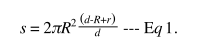Equation for the s in terms of the percentage of the area of the sphere is as follows.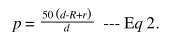As shown in Eq 2, the percentage of the spherical surface area exposed towards the passive object is dependent on the distance between the two spherical objects and the radii of the active and passive objects. If we change the radius of object A from 10 units to zero by keeping the values for r and d as 10 and 20 units respectively, the value for p will increase as the object A approaches towards a point size.

As the LEO decreases in size, the intensity of light emitting from its surface will increase and at the same time percentage of the surface area of the active object exposed towards the passive object will also increase; ultimately increasing the amount of light reaching the passive object as the active object decreases in size. When the two objects were close to each other, because the two spheres were equal in size, one full hemisphere or 50% of the surface area of each sphere gets exposed to the other. As the active object decreases in size, more percentage of the surface area of the active object gets exposed towards the passive object. When the active object turns to as close as a point size sphere, 75% of its surface area gets exposed towards the passive object. Increased exposure of the active object sheds additional amount of light on the passive objet. According to the Inverse square law, even if the LEO collapses to a point size, the amount of light reaches the passive object remains the same as when the two objects were close to each other. The ISL is based on the assumption that a same amount of the percentage of the surface area of the LEO gets exposed to the passive object irrespective of the size of the active object. In reality, more percentage of the area of the active object gets exposed to the passive object, resulting in an increase in the amount of light reaches the passive object when the size of the active object was decreased.

Amount of Light from a True Point Size Light Source

A spherical light source emanates the light in all directions but we can only see the part of the light that is emanating in our direction from that object. At any point of time, an area of maximum one hemisphere of the light emitting object, like our sun, will be visible from an external point. The other half of of the sphere will be away from the passive point therefore the light from that part of the object will never reach the external point because the object itself will obstruct the light emanating from the other half of the object towards the external point. As long as the object remains as an opaque and spherical in shape, even if it collapses to a point size, the external point receives only half the amount of light emanating from the object. If the sun has a transparent interior, we will receive the light emanating from the other half of its surface as well. It means, the earlier obstructed part of the light gets exposed when it becomes a transparent object.

When all the light emitting points on the surface of the LEO merge into a single point then there will not be anything obstructing between any of the light emanating from the LEO and the external point.Therefore, all the light emanating from all the points on the surface of the object will reach the external point. It is equal to merging all the light emitting points on the surface of the sun into a single point, a true point size LEO; similar to merging all the light bulbs on the surface of the sun into a single bulb. The intensity of the new point towards any direction will be equal to the combined intensity of all the points on the surface.

If the sun ever becomes a true point size object, it will shine very brighter than it is visible at this time. It will be like peeling the entire surface of the sun and spreading it wide open in the sky facing towards the earth. The other side of the spread out sun will also shine with equal intensity. Even though each point on the present sun spreads the light in all directions, the light spreading towards the center will never be visible. By spreading its surface or converting it to a true point size, the light spreading inwards also gets exposed.

There will not be any surface area for a true point size LEO. Lines extended for all the light emanating from a true point will be going through the center of the point. The amount of light reaches an external object from a true point LEO depends upon the angle of projection the external object makes on to the point. An area of exposure of a true point light source on to a flat surface wii increase four times if we double the distance between the objects.

Consider two flat concentric circles with the radii of 5 and 10 units for inner and outer circles respectively. In this case, the area of the outer circle will be four times bigger than the inner circle. If we focus a torch light, with a fixed angle of projection, completely on to the surface of the inner circle from a distance of 10 units and then refocus the same torch on the surface at double the initial distance (20 units) , then the light from the torch will completely occupy the outer circle. In this case, the amount of light received by the inner circle will become one fourth of the initial value, but the amount of light reaches the outer circle will remain the same in both the instances because none of the light from the torch is falling outside the outer circle. Light not necessarily be uniformly distributed all over the surface of the object in calculating the total amount of light on its surface. If we further move away the torch from the surface and increase the distance by four times of the initial value (40 units) then the amount of light reaches the outer circle will become one fourth of the light from the torch. Therefore, we can say that the ISL applies in calculating the amount of light exposed by a true point size LEO onto a flat surface only when that surface is completely exposed to the light source and then the light source was moved away from the surface.

The case of the amount of light reaches a sphere from an external true point light source is similar to the above concentric circles. A sphere can be assumed as a stack of flat and thin circular disks facing an external point. Light from an external point falls on the edges of different disks depending upon the distance between the point and the sphere. The area of exposure on the surface of the sphere forms as a spherical cap . The area covered by the cap can be expressed using the Eq. 3 where the s is the area of the cap, R is the radius of the sphere and the d is the distance between the external point and the center of the sphere.

Amount of the surface area of a sphere exposed towards a true point light source varies with the distance, thereby changing the amount of light reaches each of the completely exposed disk in the sphere until the exposed surface area is equal to one complete hemisphere of the passive object. From that point onwards, the amount of light reaches the sphere will be inversely proportional to the distance between the objects.

If we assume the radius of the sphere as 10 units then the graph for the surface area of the cap of the sphere is shown as in Fig 2. As shown in the graph, the difference in the area of the successive caps will be more when the point is closure to the sphere. As the point moves away from the sphere, the area of the cap will be close to the area of one complete hemisphere. As we can see in the graph, the area of the spherical cap exposed by the external point will never be equal to the area of one complete hemisphere but it will be close to its value at far away from the point. The difference between the amount of light calculated using the ISL and the actual amount received by the sphere will be less when the difference between the area of one hemisphere and the area of the exposed spherical cap decreases.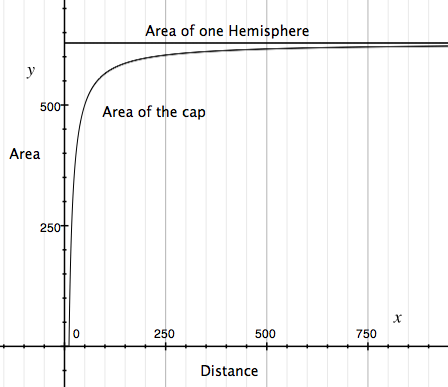Fig 2. Exposure of surface between a point and a sphere

Limitations of Inverse Square Law for Light

There are three possible scenarios for any light emitting object; a huge spherical object like our sun, a point size spherical object formed from the collapse of the same object and a true point size object. From the above analysis, it is now evident that a passive object receives more light from an active object when the object is a spherical point size LEO than it is a huge object. The same passive object will receive even greater amount of light when the LEO collapses to a true point size object. It is important to note that all of these three objects emanates the same amount of light. Variation in the amount of light received by an external passive object kept at a same distance from different forms of the same LEO violates the ISL for light. The root cause for the ISL to be false is the assumption of all the light emitting objects as a true point size objects in its derivation. A spherical and a true point size light emitting objects are not the same in the manner in which they spread the light around them. A true point size LEO spreads more light compared to any other spherical object even though the total amount of light generated by these objects are the same.

Therefore, we can't assume a spherical object as a true point size object without doubling the intensity of the object, at the same time, we can't equate a true point size object to a spherical object without halving the intensity of the object in determining the amount of light that object spreads around its surroundings. ISL is not applicable for the spherical objects, either passive or active, where the amount of the surface area exposed to another object varies with the distance between them thereby altering the amount of light received by the passive object. ISL only applies to a scenario where the initial exposure of a true point size LEO is on the whole surface of the passive object. There shouldn't be any change in the amount of the surface area of the passive object exposed towards the active object when the distance between them was either increased or decreased.

Amount of Light to An External Point

Amount of light (l ) an external point receives from a LEO depends upon the total amount of light (L) of the LEO, total surface area of the LEO (S), the distance between the center of the LEO to the external point (d) and the surface area of the LEO (s) exposed towards the external point.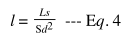Amount of light received b the external point will increase as the amount of the surface area of the LEO exposed towards the external point increases.

If the LEO is a true point size object then the surface area of the LEO exposed towards the external point will be equal to the total surface area of the LEO, therefore the amount of light received by the external point will be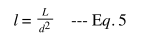Conclusion

In summary, we can conclude that inverse square law of distance for light works only when the active light emitting object is of a true point size object and the passive object is a flat two dimensional surface. In all other scenarios, the amount of light reaches a passive object depends upon the size and shape of both the active and passive objects along with the distance between them.